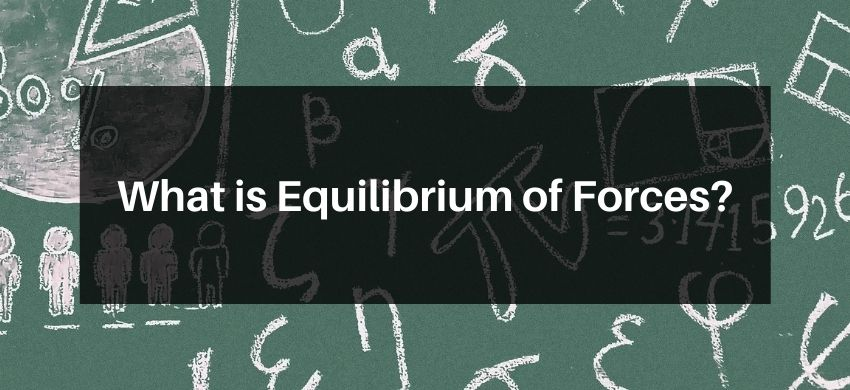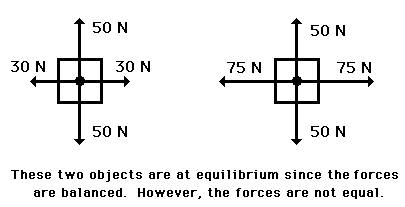Most Affordable JEE | NEET | 8,9,10 Preparation by Kota's Top IITian Doctor Faculties

# What is Equilibrium of Forces?`

## Equilibrium of Forces

Forces that have zero linear resultant and zero turning effect will not cause any change in the motion of the object to which they are applied. Such forces (and the object) are said to be in equilibrium.

Mathematically a body is said to be in equilibrium if

(a) Net force acting on it is zero, i.e., $\vec{F}_{\text {net }}=0$

(b) Net moments of all the forces acting on it about any axis is zero. Physically the body at rest is said to be in equilibrium if it is permanently at rest (unless some other force is applied on it, may disturb its equilibrium). If a body is at rest just for a moment, it does not mean it is in equilibrium.

For example, when a ball is thrown upwards, at the highest point of its journey it momentarily comes at rest, but there it is not in equilibrium. A net force (equal to its weight) is acting downward. Due to that force only it moves downwards.

Consider the two objects pictured in the force diagram shown below. Note that the two objects are at equilibrium because the forces that act upon them are balanced; however, the individual forces are not equal to each other. The 50 N force is not equal to the 30 N force.